Test: Dimensional & Model Analysis - 2

# Test: Dimensional & Model Analysis - 2 - Mechanical Engineering

Test Description

## 10 Questions MCQ Test Fluid Mechanics for Mechanical Engineering - Test: Dimensional & Model Analysis - 2

Test: Dimensional & Model Analysis - 2 for Mechanical Engineering 2023 is part of Fluid Mechanics for Mechanical Engineering preparation. The Test: Dimensional & Model Analysis - 2 questions and answers have been prepared according to the Mechanical Engineering exam syllabus.The Test: Dimensional & Model Analysis - 2 MCQs are made for Mechanical Engineering 2023 Exam. Find important definitions, questions, notes, meanings, examples, exercises, MCQs and online tests for Test: Dimensional & Model Analysis - 2 below.
Solutions of Test: Dimensional & Model Analysis - 2 questions in English are available as part of our Fluid Mechanics for Mechanical Engineering for Mechanical Engineering & Test: Dimensional & Model Analysis - 2 solutions in Hindi for Fluid Mechanics for Mechanical Engineering course. Download more important topics, notes, lectures and mock test series for Mechanical Engineering Exam by signing up for free. Attempt Test: Dimensional & Model Analysis - 2 | 10 questions in 30 minutes | Mock test for Mechanical Engineering preparation | Free important questions MCQ to study Fluid Mechanics for Mechanical Engineering for Mechanical Engineering Exam | Download free PDF with solutions
 1 Crore+ students have signed up on EduRev. Have you?
Test: Dimensional & Model Analysis - 2 - Question 1

### A 1.0 m long model of a ship is tnwed in a towing tank at a speed of 81 cm/s. To what speed of the ship of 64 m long does this correspond?

Detailed Solution for Test: Dimensional & Model Analysis - 2 - Question 1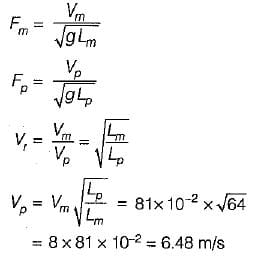Test: Dimensional & Model Analysis - 2 - Question 2

### A model boat, 1/100 size of its prototype has 0.12 N of resistance when simulating a speed of 5 m/s of the prototype. Water is the fluid in both cases. What is the corresponding resistance in the prototype? [The frictional forces can be neglected]

Detailed Solution for Test: Dimensional & Model Analysis - 2 - Question 2

The resistance offered at the free surface is the significant force and as such Froude model law is appropriate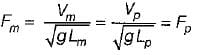If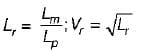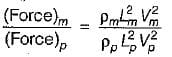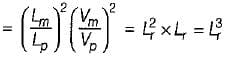∴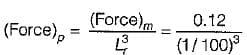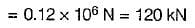Test: Dimensional & Model Analysis - 2 - Question 3

### Oil of density 917 kg/m3 and dynamic viscosity 0.29 Pa.s flows in a pipe of diameter 15 cm at a velocity of 2 m/s. What would be the velocity of flow of water in a 1 cm diameter pipe to make the two flows dynamically similar? The density and viscosity of water can be taken as 998 kg/m3 and 1.31 x 10-3 Pa.s respectivley.

Detailed Solution for Test: Dimensional & Model Analysis - 2 - Question 3

In this case, Reynolds similarity law is applicable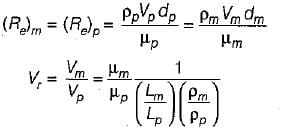Referring to oil with a subscript p and water with a suffix m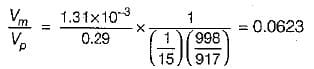∴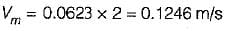Test: Dimensional & Model Analysis - 2 - Question 4

A 1 :5 scale model of a passenger car is tested in wind tunnel. The prototype velocity is 50 km/h. if the model drag is 200 N, what is the power required to overcome the drag in the prototype? The air in the model and prototype can be assumed to have the same properties.

Detailed Solution for Test: Dimensional & Model Analysis - 2 - Question 4

Reynolds similarity law is applicable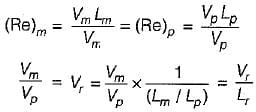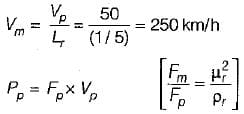∵ air is having same properties,
(Fm/Fp) - 1
∴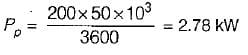Test: Dimensional & Model Analysis - 2 - Question 5

A pipe of diameter 1.5 m is required to transport an oil of relative density 0,9 and kinematic viscosity = 3 x 10-2 stoke at a rate of 3 m3/s. if a 15 cm diameter pipe with water at 20°C (n = 0.01 stoke) is used to model the above flow, the discharge in the model is

Detailed Solution for Test: Dimensional & Model Analysis - 2 - Question 5

The Reynolds number must be the same in the model and prototype for similar pipe flows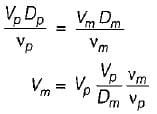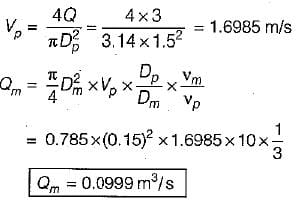Test: Dimensional & Model Analysis - 2 - Question 6

Both Reynolds number and Froude number assume, significance in one of the following example:

Test: Dimensional & Model Analysis - 2 - Question 7

Match List-I (Parameter) with List-II (Scale ratio Froude Laws) the following: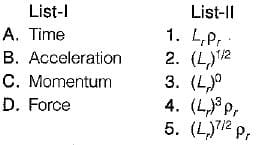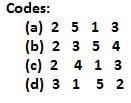Detailed Solution for Test: Dimensional & Model Analysis - 2 - Question 7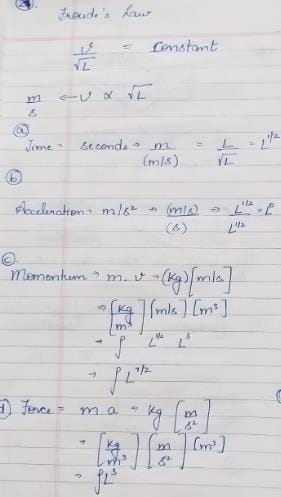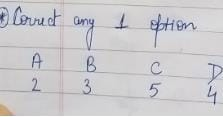Test: Dimensional & Model Analysis - 2 - Question 8

Match the List-I (Parameter) with List-ll (Scale ratios for Reynolds laws):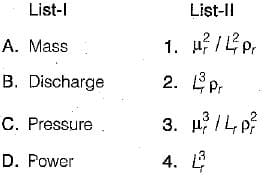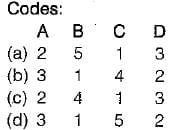Test: Dimensional & Model Analysis - 2 - Question 9

Which one of the dimensionless numbers identifies the compressibility effect of a fluid

Detailed Solution for Test: Dimensional & Model Analysis - 2 - Question 9

At higher Mach number the compressibility effect is predominant.

Test: Dimensional & Model Analysis - 2 - Question 10

Geometric similarity between model and prototype means the similarity of

Detailed Solution for Test: Dimensional & Model Analysis - 2 - Question 10

Geometric similarity ⇒ Similarity in linear dimensions.

## Fluid Mechanics for Mechanical Engineering

50 videos|90 docs|76 tests
Information about Test: Dimensional & Model Analysis - 2 Page
In this test you can find the Exam questions for Test: Dimensional & Model Analysis - 2 solved & explained in the simplest way possible. Besides giving Questions and answers for Test: Dimensional & Model Analysis - 2, EduRev gives you an ample number of Online tests for practice

## Fluid Mechanics for Mechanical Engineering

50 videos|90 docs|76 tests